Test: Diode Circuits - 2

# Test: Diode Circuits - 2

Test Description

## 15 Questions MCQ Test GATE Electrical Engineering (EE) 2023 Mock Test Series | Test: Diode Circuits - 2

Test: Diode Circuits - 2 for Electronics and Communication Engineering (ECE) 2023 is part of GATE Electrical Engineering (EE) 2023 Mock Test Series preparation. The Test: Diode Circuits - 2 questions and answers have been prepared according to the Electronics and Communication Engineering (ECE) exam syllabus.The Test: Diode Circuits - 2 MCQs are made for Electronics and Communication Engineering (ECE) 2023 Exam. Find important definitions, questions, notes, meanings, examples, exercises, MCQs and online tests for Test: Diode Circuits - 2 below.
Solutions of Test: Diode Circuits - 2 questions in English are available as part of our GATE Electrical Engineering (EE) 2023 Mock Test Series for Electronics and Communication Engineering (ECE) & Test: Diode Circuits - 2 solutions in Hindi for GATE Electrical Engineering (EE) 2023 Mock Test Series course. Download more important topics, notes, lectures and mock test series for Electronics and Communication Engineering (ECE) Exam by signing up for free. Attempt Test: Diode Circuits - 2 | 15 questions in 45 minutes | Mock test for Electronics and Communication Engineering (ECE) preparation | Free important questions MCQ to study GATE Electrical Engineering (EE) 2023 Mock Test Series for Electronics and Communication Engineering (ECE) Exam | Download free PDF with solutions
 1 Crore+ students have signed up on EduRev. Have you?
Test: Diode Circuits - 2 - Question 1

### In the question a circuit and a waveform for the input voltage is given. The diode in circuit has cutin voltage Vγ = 0. Choose the option for the waveform of output voltage vo :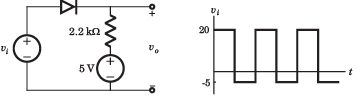​ ​ ​

Detailed Solution for Test: Diode Circuits - 2 - Question 1
• Diode is off for vi  < 5 V. Hence vo = 5 V.
• For vi > 5 V, vo = vi .Therefore (D) is correct option.
Test: Diode Circuits - 2 - Question 2

### In the question a circuit and a waveform for the input voltage is given. The diode in circuit has cutin voltage Vγ = 0. Choose the option for the waveform of output voltage vo: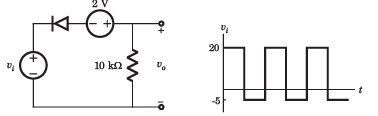Detailed Solution for Test: Diode Circuits - 2 - Question 2
• Diode will be off if vi + 2 > 0.Thus vo = 0
• For v+ 2  < 0 V, vi  < -2, vo = vi + 2 = -3V
• Thus (C) is correct option
Test: Diode Circuits - 2 - Question 3

### In the question a circuit and a waveform for the input voltage is given. The diode in circuit has cutin voltage Vγ = 0. Choose the option for the waveform of output voltage vo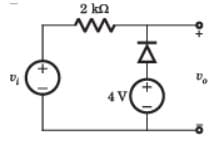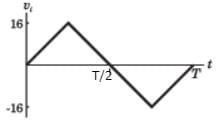Detailed Solution for Test: Diode Circuits - 2 - Question 3
• For vi  < 4 V the diode is ON and output vo = 4 V.
• For vi  >4 V diode is off and output vo = vi.
• Thus (D) is correct option.
Test: Diode Circuits - 2 - Question 4

In the question a circuit and a waveform for the input voltage is given. The diode in circuit has cutin voltage Vγ = 0. Choose the option for the waveform of output voltage vo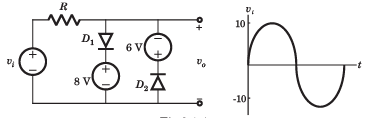Detailed Solution for Test: Diode Circuits - 2 - Question 4
• During positive cycle when vs < 8V both diode are OFF vo = vs . For vs > 8V , vo = 8V , D1 is ON.
• During negative cycle when |vs| < 6 V, both diode are OFF, vo = vs .
• For |vs| > 6V , D2 is on v0 = -6V .Therefore (C) is correct.
Test: Diode Circuits - 2 - Question 5

For the circuit in fig. P.3.1.5, let cutin voltage Vγ = 0.7 V. The plot of vo verses vi for -10 ≤ vi ≤10 V is: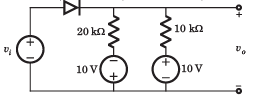Detailed Solution for Test: Diode Circuits - 2 - Question 5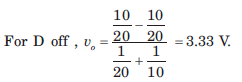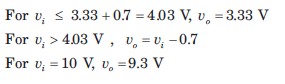Test: Diode Circuits - 2 - Question 6

The circuit inside the box in fig. contains only resistor and diodes. The terminal voltage vo is connected to some point in the circuit inside the box. The largest and smallest possible value of vo most nearly to is respectively.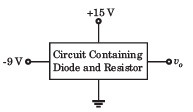Detailed Solution for Test: Diode Circuits - 2 - Question 6

The output voltage cannot exceed the positive power supply voltage and cannot be lower than the negative power supply voltage.

Test: Diode Circuits - 2 - Question 7

In the voltage regulator circuit in fig. the maximum load current iL that can be drawn is: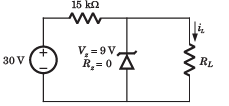Detailed Solution for Test: Diode Circuits - 2 - Question 7

At regulated power supply: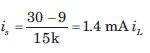will remain less than 1.4 mA.

Test: Diode Circuits - 2 - Question 8

In the voltage regulator shown in fig. the power dissipation in the Zener diode is: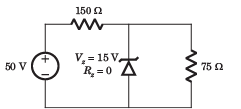Detailed Solution for Test: Diode Circuits - 2 - Question 8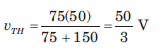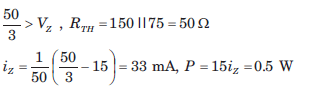Test: Diode Circuits - 2 - Question 9

The Q-point for the Zener diode in fig. is: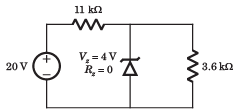Detailed Solution for Test: Diode Circuits - 2 - Question 9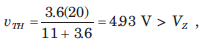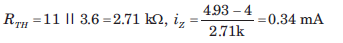Test: Diode Circuits - 2 - Question 10

In the voltage regulator circuit in fig  the power rating of Zener diode is 400 mW. The value of RL that will establish maximum power in Zener diode is: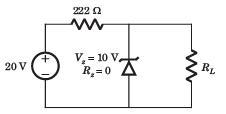Detailed Solution for Test: Diode Circuits - 2 - Question 10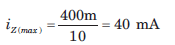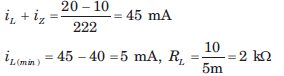Test: Diode Circuits - 2 - Question 11

In the voltage regulator circuit in fig.  the Zener diode current is to be limited to the range 5 ≤ iz ≤100 mA.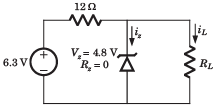The range of possible load current is:

Detailed Solution for Test: Diode Circuits - 2 - Question 11

Current through 12 Ω resistor is: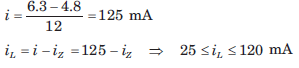Test: Diode Circuits - 2 - Question 12

In the voltage regulator circuit in fig.  the Zener diode current is to be limited to the range 5 ≤ iz ≤100 mA.The range of possible load resistance is:

Detailed Solution for Test: Diode Circuits - 2 - Question 12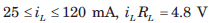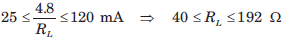Test: Diode Circuits - 2 - Question 13

In the voltage regulator circuit in fig.  the Zener diode current is to be limited to the range 5 ≤ iz ≤100 mA.The power rating required for the load resistor is:

Detailed Solution for Test: Diode Circuits - 2 - Question 13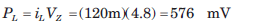Test: Diode Circuits - 2 - Question 14

The secondary transformer voltage of the rectifier circuit shown in fig. is vs = 60 sin 2π 60tV. Each diode has a cut in voltage of Vγ = 0.6 V. The ripple voltage is to be no more than Vrip = 2 V. The value of filter capacitor will be: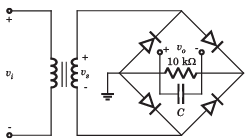Detailed Solution for Test: Diode Circuits - 2 - Question 14

vs = 60 sin 2π 60tV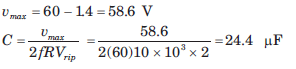Test: Diode Circuits - 2 - Question 15

The input to full-wave rectifier in fig. is vi = 120 sin2π60t V. The diode cutin voltage is 0.7 V. If the output voltage cannot drop below 100 V, the required value of the capacitor is: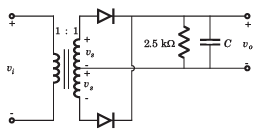Detailed Solution for Test: Diode Circuits - 2 - Question 15

Full wave rectifier: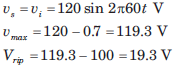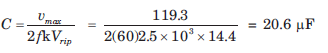## GATE Electrical Engineering (EE) 2023 Mock Test Series

21 docs|271 tests
 Use Code STAYHOME200 and get INR 200 additional OFF Use Coupon Code
Information about Test: Diode Circuits - 2 Page
In this test you can find the Exam questions for Test: Diode Circuits - 2 solved & explained in the simplest way possible. Besides giving Questions and answers for Test: Diode Circuits - 2, EduRev gives you an ample number of Online tests for practice

## GATE Electrical Engineering (EE) 2023 Mock Test Series

21 docs|271 tests